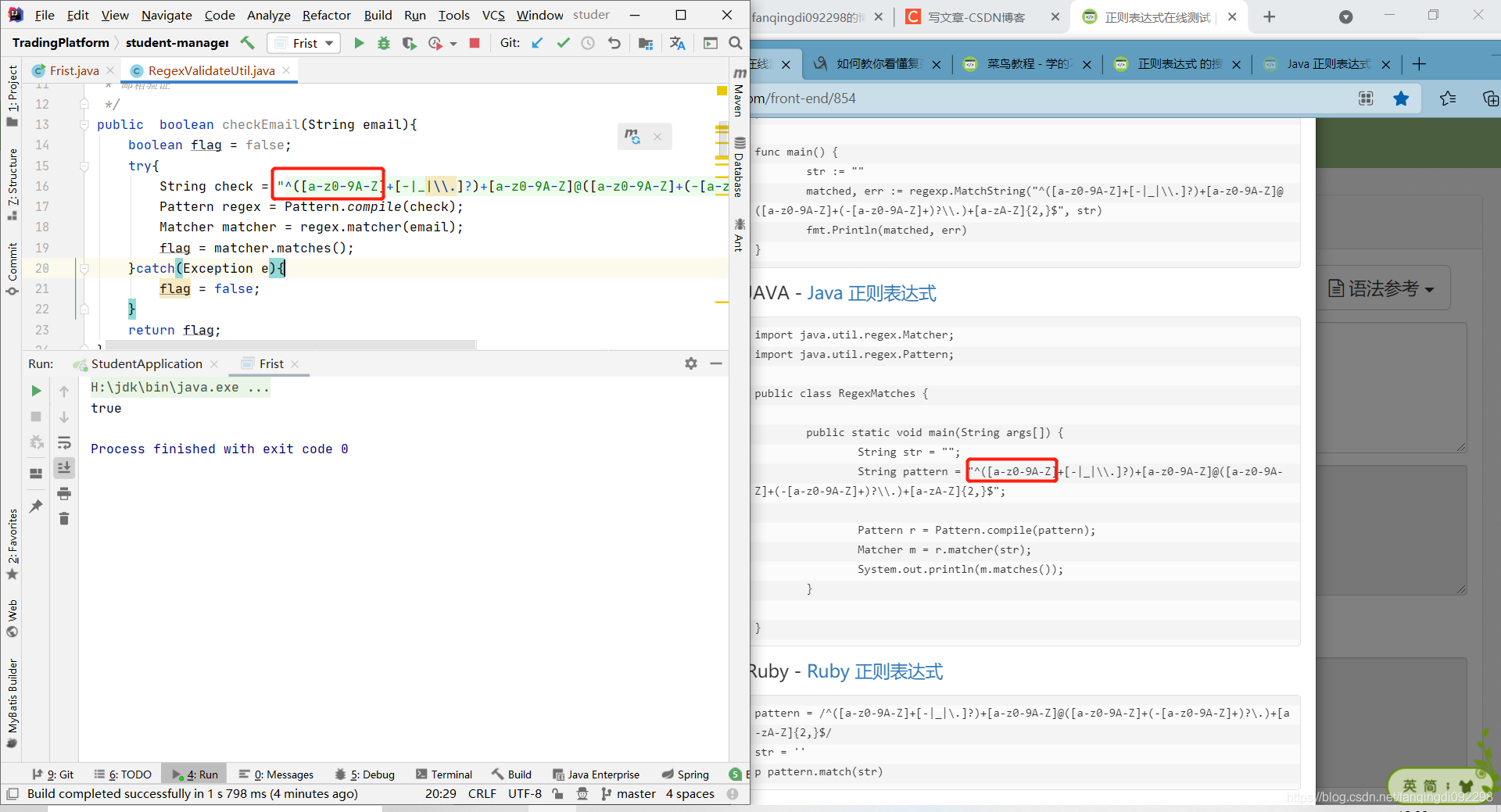# Java常用工具有哪些

### 1：生成纯数字的随机数

```package com.lucun.student.utils;

import java.util.Random;
//纯数字验证码
public class GetRandom {
public static String getFourRandom(){
Random random = new Random();
String fourRandom = random.nextInt(10000) + "";
int randLength = fourRandom.length();
if(randLength<6){//这个6代表生成6位随机数
for(int i=1; i<=6-randLength; i++)
fourRandom = "0" + fourRandom ;
}
return fourRandom;
}
}```### 2：生成包含字母的随机数（大小写）

```package com.lucun.student.utils;

import java.util.Random;

public class GetRandomEnglishMath {
public static String getCharAndNumr(int length) {
String val = "";
Random random = new Random();
for (int i = 0; i < length; i++) {
String charOrNum = random.nextInt(2) % 2 == 0 ? "char" : "num";
if ("char".equalsIgnoreCase(charOrNum)) {
// 取得大写字母还是小写字母
int choice = random.nextInt(2) % 2 == 0 ? 65 : 97;
val += (char) (choice + random.nextInt(26));
}else if ("num".equalsIgnoreCase(charOrNum)) { // 数字
val += String.valueOf(random.nextInt(10));
}
}
return val;
}
}```### 3：正则表达式验证手机号（2021年期间的手机号）

`"^([a-z0-9A-Z]+[-|_|\\.]?)+[a-z0-9A-Z]@([a-z0-9A-Z]+(-[a-z0-9A-Z]+)?\\.)+[a-zA-Z]{2,}\$"`